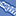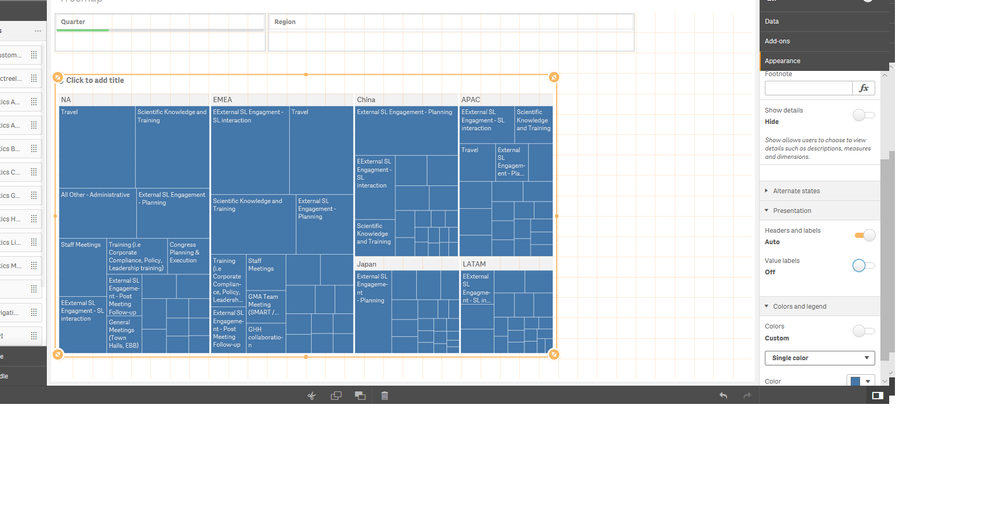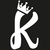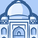# New to Qlik Sense

If you’re new to Qlik Sense, start with this Discussion Board and get up-to-speed quickly.

Announcements
Our next Qlik Insider session will cover new key capabilities. Join us August 11th REGISTER TODAY
cancel
Showing results for
Did you mean:Creator II

## If else basing on color in Treemap of Qliksense

Hi,

I have developed a Treemap where Region and Task name are dimension . Measure is hours (MSL hours) . Top 20 task has to be seen .The measures code :(sum({<FM ={'MSL'}>}Q1hours)+sum({< FM ={'MSL'}>}Q2hours)+sum({<FM ={'MSL'}>}Q3hours)+ sum({<FM ={'MSL'}>}Q4hours)

)

Task Dimension limits to top 20.

The requirement is  if Task greater than or equal to 5 % of total hours are Gold color.I wrote a color condition like below but it is not 100 percent correct. please suggest.

Thanks,

Niha

6 RepliesPartner

Hi

try like this in color expression by changing the expression according to your dimension and measures

if(Aggr(Sum(Quantity),ProductName)>=(5/100)*Aggr(Sum(Quantity),CategoryName),Green(),Red())

I have attached the chart file please go through that it will give you correct insight what you are looking for.

Hope this helps

ThanksCreator II
Author

Thanks. if  want top 2o Task, then for every task I have to write one expression, so for 20 task I have to write 20 expression , correct?ChampionCreator II
Author

Hello,

Thanks. Not Yet as my requirements changed and I need to color code according to measure .

Thanks,

NihaLuminary Alumni

Use color by expression and a color expression of:

If(Column(1) >
(sum({<FM ={'MSL'}>}TOTAL Q1hours)+sum({< FM ={'MSL'}>}TOTAL Q2hours)+sum({<FM ={'MSL'}>}TOTAL Q3hours)+ sum({<FM ={'MSL'}>}TOTAL Q4hours) * .05
,rgb(231,187,73)
,rgb(144,144,144)
)Partner

Hi,

This might help

If(Column(1) /
(sum({<FM ={'MSL'}>}TOTAL Q1hours)+sum({< FM ={'MSL'}>}TOTAL Q2hours)+sum({<FM ={'MSL'}>}TOTAL Q3hours)+ sum({<FM ={'MSL'}>}TOTAL Q4hours)) >'0.05',green(),red()
)

Regards,

PrashantTags
Community Browser